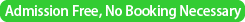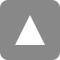﻿ 第29回統計的機械学習セミナー / The 29th Statistical Machine Learning Seminar | 統計数理研究所

# 第29回統計的機械学習セミナー / The 29th Statistical Machine Learning Seminar

Date&Time
2016年3月30日（水）13:30-
/ 30 March, 2016 (Wed) 13:30-Place

/ Seminar Room 5 (3F) @ The Institute of Statistical Mathematics【13:30-14:30】

Speaker1
Dino Sejdinovic (University of Oxford)
Title
Kernel Embeddings for Inference with Intractable Likelihoods
Abstract
Flexible representations of probability distributions using embeddings into a reproducing kernel Hilbert space (RKHS) have been used to construct powerful non-parametric hypothesis tests and association measures. In this talk, I will overview recent applications of this framework which lead to inference improvements in models with intractable likelihoods. First, a Kernel Adaptive MCMC algorithm will be introduced for the purpose of sampling from a target distribution with strongly nonlinear support. The algorithm uses the RKHS covariance of the samples to inform the choice of proposal. The procedure requires neither gradients nor any other higher order information about the target, making it attractive for exact-approximate methods such as pseudo-marginal MCMC. Second, I will overview applications of kernel embeddings in the context of Approximate Bayesian Computation (ABC). In ABC, distribution regression and conditional distribution regression from the embeddings defined on simulated data to the parameter space can be used for "semi-automatic" construction of informative summary statistics. Moreover, an effective dissimilarity criterion in Approximate Bayesian Computation (ABC) can be constructed based on RKHS distances -- maximum mean discrepancies -- between empirical distributions of observed and simulated data, obviating need for any handcrafed summary statistics in some cases.
Reference
* D. Sejdinovic, H. Strathmann, M. G. Lomeli, C. Andrieu, and A. Gretton, Kernel Adaptive Metropolis-Hastings, in International Conference on Machine Learning (ICML), 2014, pp. 1665–1673.
* M. Park, W. Jitkrittum, and D. Sejdinovic, K2-ABC: Approximate Bayesian Computation with Kernel Embeddings, in International Conference on Artificial Intelligence and Statistics (AISTATS), 2016.
* J. Mitrovic, D. Sejdinovic, and Y. W. Teh, DR-ABC: Approximate Bayesian Computation with Kernel-Based Distribution Regression, ArXiv e-prints:1602.04805, 2016.【14:40-15:40】

Speaker2
Arthur Gretton (Gatsby Computational Neuroscience Unit, University College London)
Title
Kernel Adaptive Hamiltonian Monte Carlo using the Infinite Exponential Family
Abstract
We propose Kernel Hamiltonian Monte Carlo (KMC), a gradient-free adaptive MCMC algorithm based on Hamiltonian Monte Carlo (HMC). HMC is a powerful approach to Markov Chain Monte Carlo, since it generates successive samples with low correlation even for distributions in high dimensions and with complex nonlinear support. On target densities where classical HMC is not an option due to intractable gradients, KMC adaptively learns the target's gradient structure by fitting an exponential family model in a Reproducing Kernel Hilbert Space. Our talk addresses two topics: first, we describe the properties of the exponential family model, show consistency for a wide class of target densities, and provide convergence rates under smoothness assumptions. Second, we demonstrate two strategies  to approximate the gradient of this model efficiently, and show how these approximate gradients may be used in constructing an adaptive Hamiltonian Monte Carlo method. We establish empirical performance of the kernel adaptive HMC sampler with experimental studies on both toy and real-world applications, including  exact-approximate MCMC for Gaussian process classification.【15:50-16:50】

Speaker3
Kenji Fukumizu (The Institute of Statistical Mathematics)
Title
Persistence weighted Gaussian kernel for topological data analysis
Abstract
Topological data analysis (TDA) is an emerging mathematical method for extracting topological information in multi-scale data. In the method, a persistence diagram, 2-D plot for illustrating the topology, is widely used as a descriptor of data.   Persistence diagrams provide information for distinguishing robust and noisy topological structures in data, taking multiscale look at underlying geometric structure.  In this work, we introduce a kernel method for persistence diagrams to apply statistical methods systematically to TDA.  We propose a new positive definite kernel for persistence diagrams, aiming at flexibly distinguishing significant topological structures from noise.  We also discuss a computational challenge of TDA caused by its large scale of diagrams, and propose an approximate computation method.  As a theoretical background, a stability theorem is proved, which shows that a small change of data points causes only small changes of the distance measure associated with the kernel. Finally, the proposed kernel is applied to practical problems of data analysis in materials science and biology, showing favorable results in comparison with other existing methods.
This work has been done with Genki Kusano and Yasuaki Hiraoka (Tohoku Univ.).